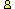CTK Exchange CTK Wiki Math Front Page Movie shortcuts Personal info Awards Terms of use Privacy Policy Cut The Knot! MSET99 Talk Games & Puzzles Arithmetic/Algebra Geometry Probability Eye Opener Analog Gadgets Inventor's Paradox Did you know?... Proofs Math as Language Things Impossible My Logo Math Poll Other Math sit's Guest book News sit's Recommend this siteCTK Exchange

 Subject: "Trigonometric proof of Pythagorean theorem (proof 84)." Previous Topic | Next Topic
 ConferencesThe CTK ExchangeThoughts and SuggestionsTopic #99Printer-friendly copyEmail this topic to a friend Reading Topic #99
Janez Lesjakguest
Feb-01-11, 03:45 PM (EST)

"Trigonometric proof of Pythagorean theorem (proof 84)."

 What is actually meant by trigonometric proof of Pythagorean theorem?Trigonometric functions are defined in analysis by infinite series. All of their properties are then derived from them, including the number Pi and the "Pythagorean relation" (sin x)(sin x) + (cos x)(cos x) = 1. The important question is how to relate the argument x to the Euclidean angle?

Alert | IP Printer-friendly page | Reply | Reply With Quote | Top
alexbCharter Member
2757 posts
Feb-01-11, 07:01 PM (EST)1. "RE: Trigonometric proof of Pythagorean theorem (proof 84)."
In response to message #0

 Far as I am concerned sine is defined as the ratio of a leg of a right triangle to its hypotenuse.I am curious why some function be called "trigonometric" if they defined in analysis by infinite series.However, you asked a question. A trigonometric proof is a proof that uses trig functions in a significant way.

Alert | IP Printer-friendly page | Reply | Reply With Quote | TopJanez Lesjakguest
Feb-08-11, 07:30 PM (EST)

2. "RE: Trigonometric proof of Pythagorean theorem (proof 84)."
In response to message #1

 OK, I know how trig functions are defined in geometry - by ratios of sides of a right angled triangle, but then the fundamental relation between sine and cosine requires Pythagorean theorem, the same holds for the addition theorems. So what was earlier - the egg or the hen?Using the trig functions in a proof of a geometric theorem actually means using theorems (and axioms) on similarity and congruence, so trig functions actually do not bring anything new to geometry. The term "trigonometric" has of course a historical origin, as a strict mathematical definition of trig functions comes from analysis (not necessarily by infinite series, there is a definition by inversion of algebraic integrals).

Alert | IP Printer-friendly page | Reply | Reply With Quote | TopalexbCharter Member
2757 posts
Feb-08-11, 07:41 PM (EST)3. "RE: Trigonometric proof of Pythagorean theorem (proof 84)."
In response to message #2

 >OK, I know how trig functions are defined in geometry - by >ratios of sides of a right angled triangle, but then the >fundamental relation between sine and cosine requires >Pythagorean theorem, the same holds for the addition >theorems. So what was earlier - the egg or the hen? I think that in mathematics this is a wrong question. There is a multitude of sentences that could be derived from each other. There is no point in asking which came first, except historically or chronologically. But this is not what we are talking about here.>Using the trig functions in a proof of a geometric theorem >actually means using theorems (and axioms) on similarity and >congruence, so trig functions actually do not bring anything >new to geometry. I believe I out forth an explanation as to why I think that proof desrves to be called "trigonometric". It is based on some identities that, although could be written in terms of similarities, gain at least in clarity or owe their existence outright to their trigonometric interpretation. Do write the sibtraction formula for sine in "geometric terms".So I believe that that proof is made significantly more transparent through the use of trigonometric functions.>The term "trigonometric" has of course a >historical origin, as a strict mathematical definition of >trig functions comes from analysis (not necessarily by >infinite series, there is a definition by inversion of >algebraic integrals). Neah, can't believe that. Etimologically, "trigonometry" means measuring a triangle.

Alert | IP Printer-friendly page | Reply | Reply With Quote | Top

 Conferences | Forums | Topics | Previous Topic | Next Topic
 Select another forum or conference Lobby The CTK Exchange (Conference)   |--Early math (Public)   |--Middle school (Public)   |--High school (Public)   |--College math (Public)   |--This and that (Protected)   |--Guest book (Protected)   |--Thoughts and Suggestions (Public) Educational Press (Conference)   |--No Child Left Behind (Public)   |--Math Wars (Public)   |--Mathematics and general education (Public)You may be curious to have a look at the old CTK Exchange archive.
Please do not post there.